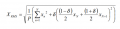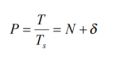# Calculating 1 cycle RMS for 50/60Hz signals

#### Blue_Electronx

Joined Jun 10, 2019
112
I found this paper to be useful. It says:

Due the characteristics of AC energy, voltage and current waveforms mean are zero; however, during abnormal operation fluctuations may occur or oftentimes the offset error introduced by transducers must be accounted for the correct characterization of the energy. These calculations are performed with a digital processing, using sequential samples with sample frequency equal to N times the AC frequency, nominally 60 Hz or 50 Hz. If the value N exactly matches the ratio between sample frequency fs = 1/Ts and AC frequency f = 1/T, there are only quantization errors due to finite length of bit number of samples. If, however, the ratio fs/f is not an integer value, what occurs most of the cases, more errors are introduced (Mesrobian et al., 1991).
So in my case for one cycle, I can't have an integer N that matches both 50 and 60 Hz and possible fluctuations. In the microcontroller, the plan I have is to set an ADC triggered by a timer for a fixed sampling frequency. I can also use DMA or double buffer approach if needed. The formula for RMS proposed in the paper iswhere N is the integer part of P, and 0≤ <1 is the fractional part.

I'm not a software/firmware guy so basically I'm asking for some help on how to code this equation in the microcontroller, like a pseudocode. Any other suggestion is welcome.

Paper: http://www.eletrica.ufpr.br/edu/artigos/CIL22-012_final_gerson.pdf

#### MrChips

Joined Oct 2, 2009
24,223
What is your sampling frequency or sampling interval?

#### crutschow

Joined Mar 14, 2008
27,737
You want the sampling rate to be a multiple integer of both 50 and 60 cycles (such as 300 samples/second).